# i4connected Knowledgebase 5.6

#### Edit and Test formulas

Abstract

Learn how to manage i4connected Measure Aggregation formulas and how to test them in real-time, using data, before applying them.

The editing and testing of formulas are done using the specialized Test Aggregation formula panel available for all measure aggregations. Here, the user can create a new formula or edit an existing one and test it against the real data, before applying it.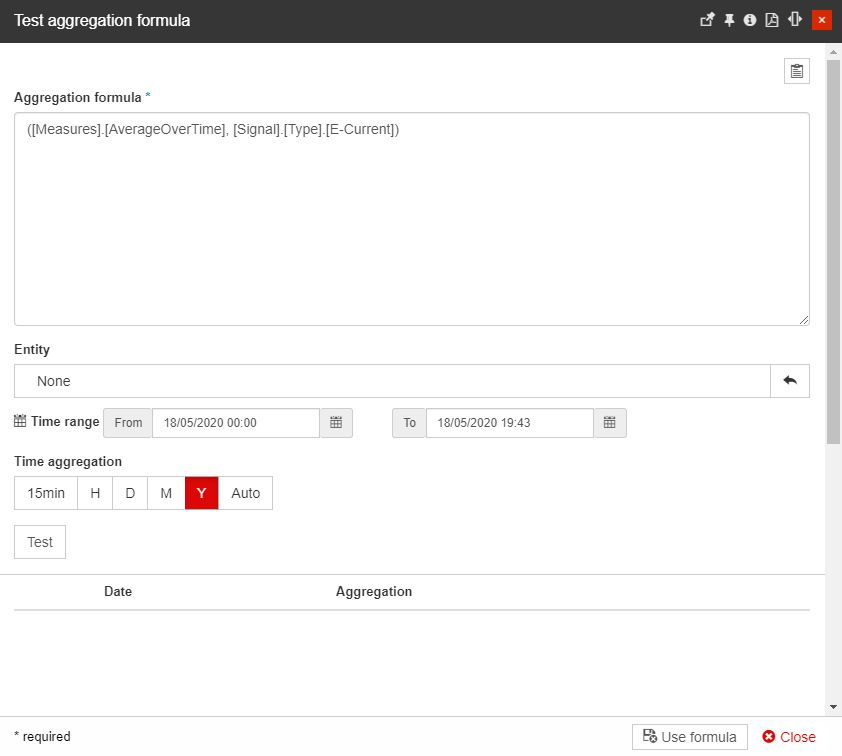The Test aggregation formula panel

The Aggregation formula field is the place where the actual formula resides. This field will be populated with the existing formula in the case of editing or will be empty in the case of creating a new formula. Besides the formula field, the user has other helpful options available:

• The MDX query snippets expand the Test aggregation formula with a set of predefined queries. The user can select these queries for further use.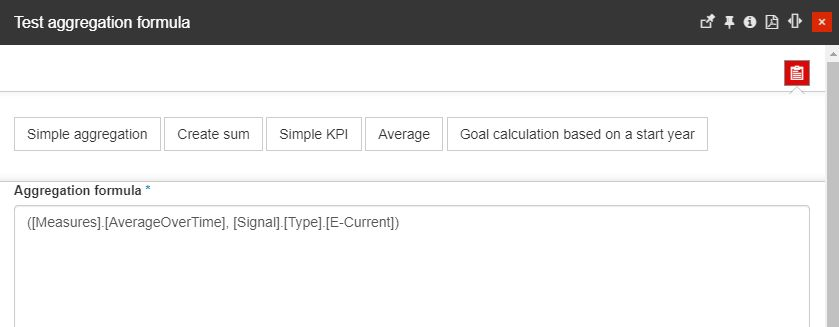The MDX Query Snippets

These Multidimensional Expressions (MDX) predefined queries already have their criteria established and are scoped for analytical processing (OLAP) using the database management system.

The syntax formulas cover only the most general calculation sets such as:

• Simple aggregation

• Create sum

• Simple KPI

• Average

• Goal calculation based on a start year

• The Entity option allows the user to select an Organizational Unit, Site, Area, or Device for which the formula will be applied in the testing scenario. The selection is done using the Object Filter panel, which allows the user to filter the existing entities by their name.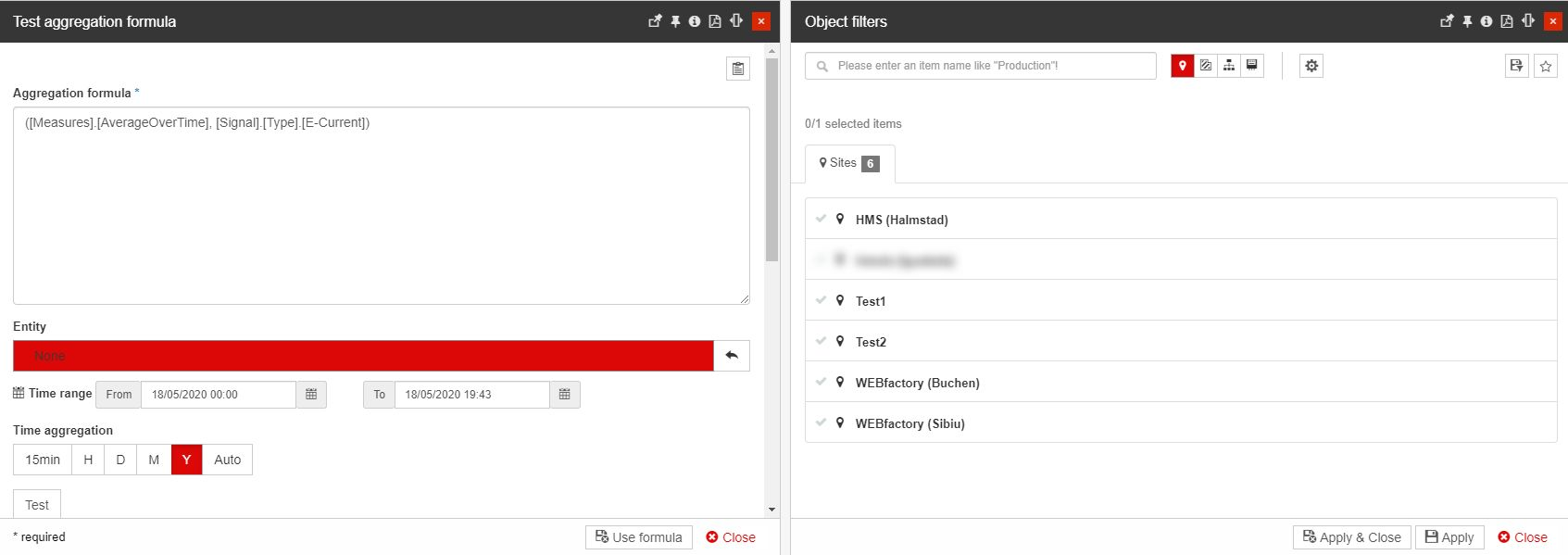Select the Entity

• The Time Range option allows the user to define a time range for which the formula will be applied. The user can define the start of the time range (From) and the end of the time range (To).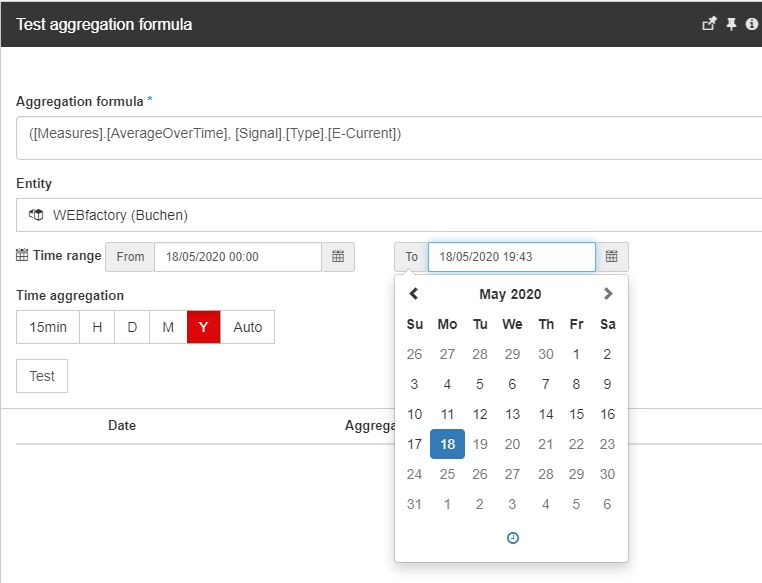Select the Time range

• The Time Aggregation option provides the user the option to select one of the predefined time periods for which the formula will be applied. The predefined options are 15 minutes, Hour, Day, Week, Month, Year, and All.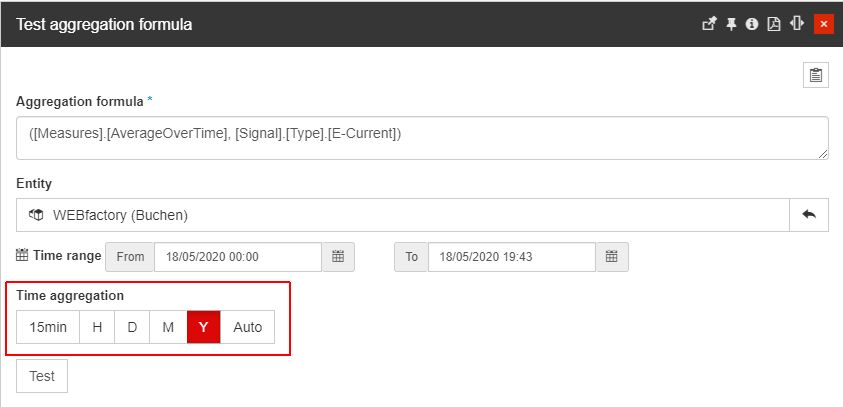Select the Time aggregation

After setting up the formula, the user can test the environment by clicking the Test button to test the formula against these options. The application will execute the request using the new formula and will display the first 10 results in the results table below.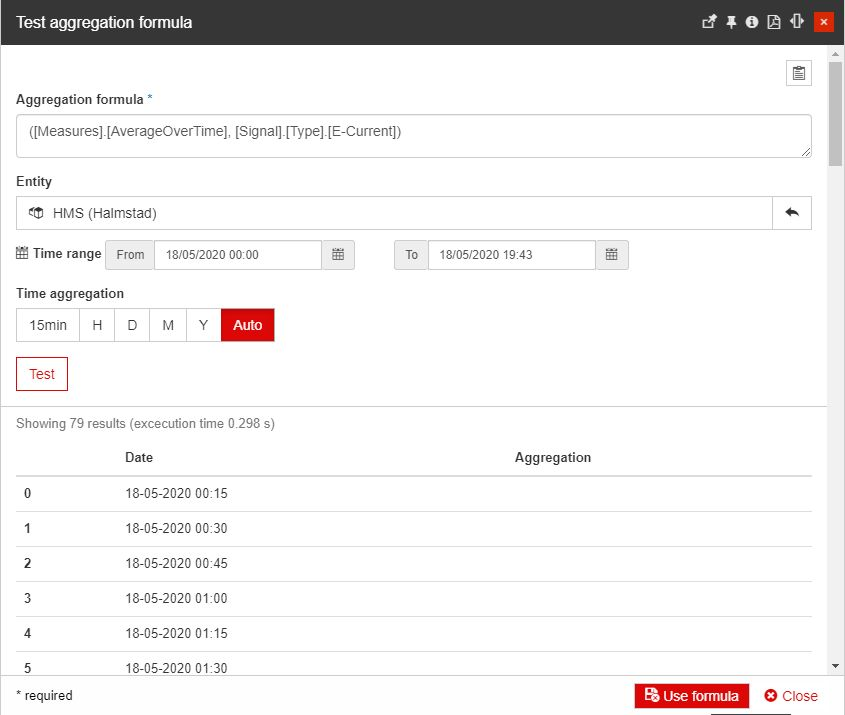Testing the aggregation formula

By clicking the Use formula button, the Test aggregations formula panel is closed and the formula is applied to the respective measure aggregation.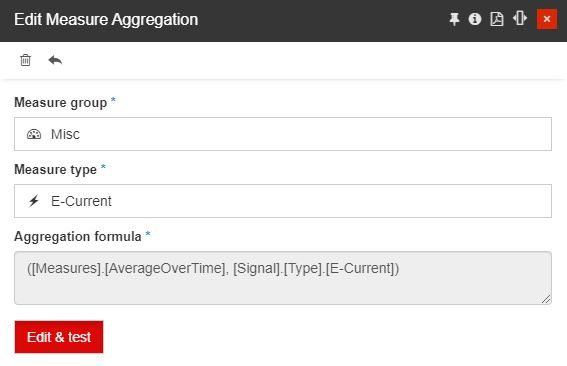Formula applied to the Measure Aggregation